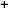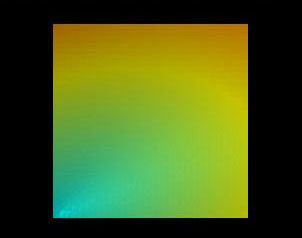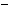Labware - MA35 Multivariable Calculus - Two Variable Calculus
 MA35 Labs 2 » Two Variable Calculus Contents2.4 Integration 2.4.2 Riemann Integral 2.4.6 Change of Variables 2.4.7 Total Mass of a Region in the Plane 2.4.8 Center of Mass 2.4.9 Moment of Inertia Search

Total Mass of a Region in the Plane (Page: 1 | 2 )

Text

The mass of an object is calculated by integrating its density function over the region of the domain that it occupies.Demos

 Density Functions and MassThe "Density: rho(x,y)" window shows the density as a color gradient, where cyan indicates rho=0, yellow rho=1, and red rho=2. The "Mass" window illustrates the mass of the object as the volume underneath the function graph of the density function rho.Examples

The Mass of an Object with Density @{rho}(x,y) = 4x + 8y@Exercises

• Will the double integral of rho over this square region find the mass of a three-dimensional object? Explain. Is there a way to modify the double integral to find the mass of a cubic region R determined by 0 {leq} x {leq} 1, 0 {leq} y {leq} 1, 0 {leq} z {leq} 1 using the same density function ρ(x,y)?
• Consider a density function of three variables. What would the triple integral of the density function represent? Could the mass be visualized as the volume underneath the function graph of the density function? What is the dimension of the space in which the function graph would exist?